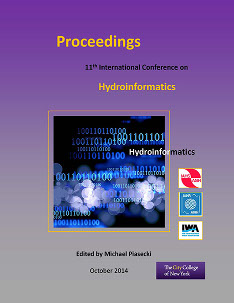## International Conference on Hydroinformatics

Presentation

8-1-2014

#### Abstract

Open-channel hydraulics’ (OCH) research traditionally links empirical formulas to observational data. One of the most common equations in OCH is Manning’s formula for open channel flow (Q) driven by gravity (also known as the Gauckler-Manning-Strickler formula). The formula relates the cross-sectional average velocity (V=Q/A), the hydraulic radius (R), and the slope of the water surface (S) with a friction coefficient n, characteristic of the channel’s surface. Here we show a practical example where Genetic Programming (GP), a technique derived from Bioinformatics, can be used to derive an empirical relationship based on different synthetic datasets of the aforementioned parameters. Specifically, we evaluated if Manning’s formula could be retrieved from datasets with 300 pentads of A, n, R, S, and Q (from Manning’s equation) using GP. The cross-validated results show success retrieving the functional form from the synthetic data and encourage the application of GP on problems where traditional empirical relationships show high biases, like sediment transport. The results also show alternative flow equations that can be used in the absence of one of the predictors and approximate Manning’s equation.